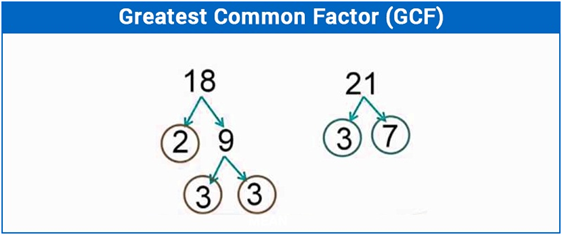# Greatest Common Factor

In Mathematics, a factor is a number which when multiplied by other numbers to get the desired numbers. The resulting number is also known as factors. Usually, the numbers can be factored into different combinations. The factors can be easily figured out if you are familiar with the multiplication tables. Here, we are going to discuss what is the greatest common factor, and how to find GCF with examples.

## What is the Greatest Common Factor?

The largest number, which is the factor of two or more number is called the Greatest Common Factor (GCF). It is the largest number (factor) that divide them resulting in a Natural number. Once all the factors of the number are found, there are few factors which are common in both. The largest number that is found in the common factors is called the greatest common factor. The GCF is also known as the Highest Common Factor (HCF)

Let us consider the example given below:For example – The GCF of 18, 21 is 3. Because the factors of the number 18 and 21 are:

Factors of 18 = 2×9 =2×3×3

Factors of 21 = 3×7

Here, the number 3 is common in both the factors of numbers. Hence, the greatest common factor of 18 and 21 is 3.

Similarly, the GCF of 10, 15 and 25 is 5.

## How to Find the Greatest Common Factor?

If we have to find out the GCF of two numbers, we will first list the prime factors of each number. The multiple of common factors of both the numbers results in GCF. If there are no common prime factors, the greatest common factor is 1.

Finding the GCF of a given number set can be easy. However, there are several steps need to be followed to get the correct GCF. In order to find the greatest common factor of two given numbers, you need to find all the factors of both the numbers and then identify the common factors.

Find out the GCF of 18 and 24

Prime factors of 18 – 2×3×3

Prime factors of 242×2×2×3

They have factors 2 and 3 in common so, thus G.C.F of 18 and 24 is 2×3 = 6

Also, try: GCF calculator

## GCF and LCM

Greatest Common Factor of two or more numbers is defined as the largest number that is a factor of all the numbers.

Least Common Multiple of two or more numbers is the smallest number (non-zero) that is a multiple of all the numbers.

Factoring Greatest Common Factor

Factor method is used to list out all the prime factors, and you can easily find out the LCM and GCF. Factors are usually the numbers that we multiply together to get another number.

Example- Factors of 12 are 1,2,3,4,6 and 12 because 2×6 =12, 4×3 = 12 or 1×12 = 12. After finding out the factors of two numbers, we need to circle all the numbers that appear in both the list.

### Greatest Common Factor Examples

Example 1:

Find the greatest common factor of 18 and 24.

Solution:

First list all the factors of the given numbers.

Factors of 18 = 1, 2, 3, 6, 9 and 18

Factors of 24 = 1, 2, 3, 4, 6, 8, 12 and 24

The largest common factor of 18 and 24 is 6.

Thus G.C.F. is 6.

Example 2:

Find the GCF of 8, 18,28 and 48.

Solution:

Factors are as follows-

Factors of 8 = 1, 2, 4, 8

Factors of 18 = 1, 2, 3, 6, 9, 18

Factors of 28 = 1, 2, 4, 7, 14, 28

Factors of 48 = 1, 2, 3, 4, 6, 8, 12, 16, 24, 48

The largest common factor of 8, 18, 28, 48 is 2. Because the factors 1 and 2 are found all the factors of numbers. Among these two numbers, the number 2 is the largest numbers. Hence, the GCF of these numbers is 2.

Stay tuned with BYJU’S – The Learning App and also visit www.byjus.com to get more Maths-related concepts to learn with ease.